# 1 神经元节点¶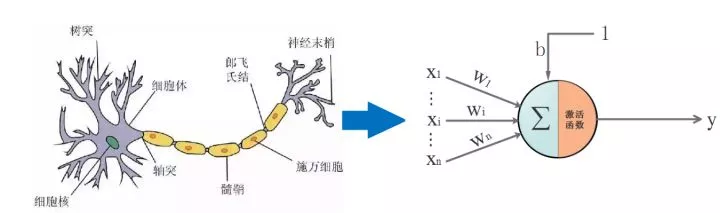（1）sigmod函数 $$f(x) = \frac{1}{{1 + {e^{ - x}}}}$$ sigmoid函数可以将整个实数范围的的任意值映射到[0,1]范围内，当输入值较大时,sigmoid将返回一个接近于1的值,而当输入值较小时，返回值将接近于0。sigmod函数图像如图2所示。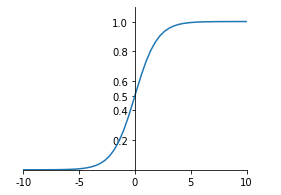（2）Relu函数 $$f(x) = \max (0,x)$$ relu（Rectified Linear Units修正线性单元），是目前被使用最为频繁得激活函数，relu函数在x<0时，输出始终为0。由于x>0时，relu函数的导数为1，即保持输出为x，所以relu函数能够在x>0时保持梯度不断衰减，从而缓解梯度消失的问题，还能加快收敛速度，还能是神经网络具有稀疏性表达能力，这也是relu激活函数能够被使用在深层神经网络中的原因。由于当x<0时，relu函数的导数为0，导致对应的权重无法更新，这样的神经元被称为"神经元死亡"。relu函数图像如图3所示。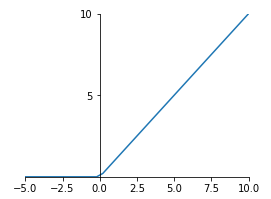（3）softmax函数 $$f({x_i}) = \frac{{{e^{{x_i}}}}}{{\sum\limits_i {{e^{{x_i}}}} }}$$ softmax函数是sigmoid函数的进化，在处理分类问题是很方便，它可以将所有输出映射到成概率的形式，即值在[0,1]范围且总和为1。例如输出变量为[1.5,4.4,2.0]，经过softmax函数激活后，输出为[0.04802413, 0.87279755, 0.0791784 ],分别对应属于1、2、3类的概率。

# 2 前馈神经网络¶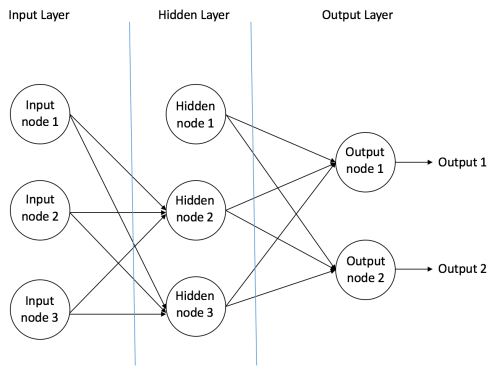（1）输入层节点。输入层节点是前馈神经网络的第一层节点，作为外部数据到神经网络的入口而存在，输入层节点不负责进行任何技术，只是将外部输入的数据传递到隐藏层节点。
（2）隐藏层节点。隐藏层节点是前馈神经网络的中间部分，它不与外界进行直接接触（这也是为什么成为隐藏层的原因）。隐藏层节点负责对输出层节点传递过来的数据进行一定运算，然后传递给输出层。前馈神经网络可以零个或多个隐藏层。
（3）输出层节点。输出层节点是整个前馈神经网络结构上最末尾上一层的节点，负责将隐藏层中传递过来的信息进行整合并输出到外界。

（1）单层感知机模型。单层感知机模型是最简单的一种神经网络模型，不包含隐藏层，也只能处理线性任务。关于单层感知机模型，请参考我的另一篇博客
（2）多层感知机。多层干自己是单程感知机的进化版，其组成结构中至少包含一个隐藏层，且在层与层之间添加了激活函数进行非线性变换，使其可以适用于非线性任务中。下文中，我们将围绕多层感知机对神经网络展开介绍。

# 3 多层感知机¶

（1）输入层。输入层具有三个节点，偏置节点的值为$1$(有时候偏置并不会作为一个节点，而是作为下一层节点中的一个参数而存在)，其它两个节点将$X1$和$X2$作为外部输入（其数值取决于输入数据集）。如上所述，在输入层中不执行任何计算，因此输入层中节点的输出分别为$1$、$X1$和$X2$，它们被馈送到隐藏层。
（2）隐藏层。图5所示网络结构隐藏层中同样具有三个节点，其偏置节点的输出为1，隐藏层中其他两个节点的输出取决于输入层$（1，X1，X2）$的输出以及与之相关的权值，权值与$（1，X1，X2）$运算后使用激活函数$f$进行非线性变换，最终的结果作为输出传递到下一层。
（3）输出层。输出层具有两个节点，这两个节点从隐藏层获取输入，并执行与红色隐藏层节点所示的类似计算，计算结果（Y1和Y2）用作为多层感知器的输出。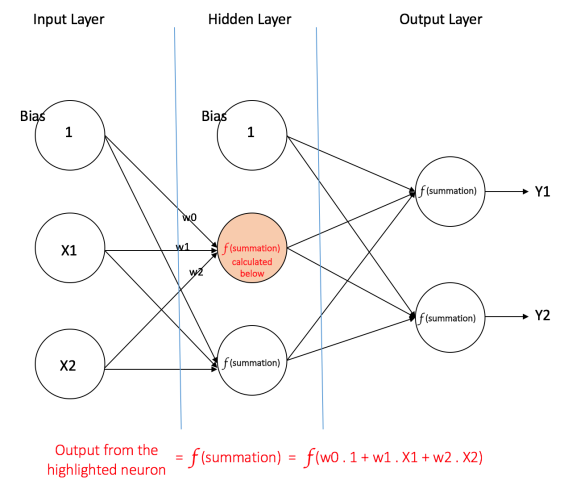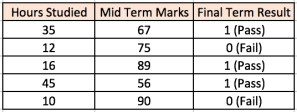# 4 反向传播算法¶

(1)特征向量[35, 67]
(2)目标向量[1, 0]

$$V = f(1 \times w1 + 35 \times w2 + 67 \times w3)$$ 隐藏层中，另外两个节点的输出计算也是类似的，只不过在图中并没有表示出来。当三个隐藏层节点完成计算后，获得的结果将继续往下一层也就是输出层传输，输出层将输出两个概率值。假设输出层中两个节点的输出概率分别为0.4和0.6（由于权重是随机分配的，因此输出也将是随机的）。我们可以看到，计算出的概率（0.4和0.6）与期望的概率（分别为1和0）相距甚远，误差较大，不过到此第一次的前向传播就完成了。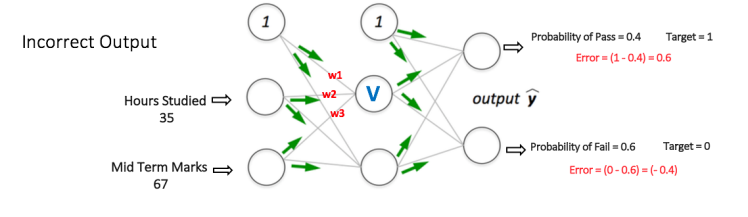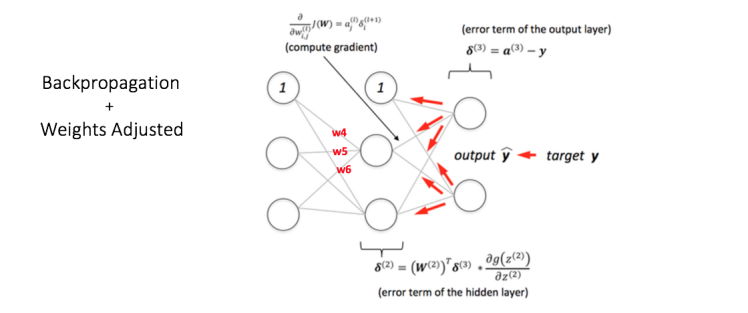# 5 Tensorflow实现人工神经网络¶

In :
import tensorflow as tf
import matplotlib.pyplot as plt
from tensorflow import keras
from tensorflow.keras import datasets, layers, optimizers, Sequential ,metrics


In :
(x, y), (x_test, y_test) = datasets.fashion_mnist.load_data()


In :
index = 1
fig, axes = plt.subplots(4, 3, figsize=(8, 4), tight_layout=True)
for row in range(4):
for col in range(3):
axes[row, col].imshow(x[index])
axes[row, col].axis('off')
axes[row, col].set_title(y[index])
index += 1
plt.show()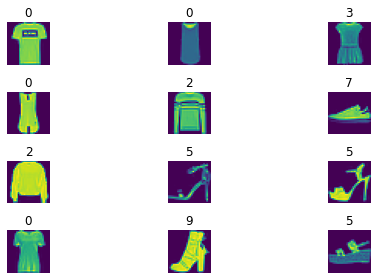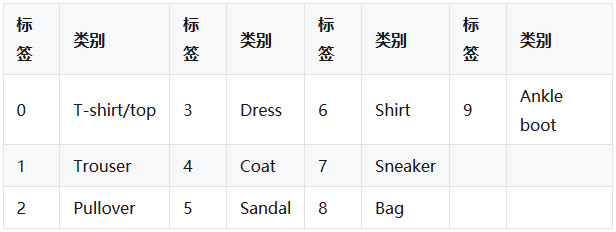In :
def preprocess(x, y):
x = tf.cast(x, dtype=tf.float32) / 255.
y = tf.cast(y, dtype=tf.int32)
return x, y

In :
print(x.shape, y.shape)
print(x_test.shape, y_test.shape)


(60000, 28, 28) (60000,)
(10000, 28, 28) (10000,)

In :
batchs = 128  # 每个簇的大小


In :
db = tf.data.Dataset.from_tensor_slices((x, y))
db = db.map(preprocess).shuffle(10000).batch(batchs)


In :
db_test = tf.data.Dataset.from_tensor_slices((x_test, y_test))
db_test = db_test.map(preprocess).batch(batchs)


In :
model = Sequential([
layers.Dense(256, activation=tf.nn.relu),  #  [b, 784]  --> [b, 256]
layers.Dense(128, activation=tf.nn.relu),  #  [b, 256]  --> [b, 128]
layers.Dense(64, activation=tf.nn.relu),  #  [b, 128]  --> [b, 64]
layers.Dense(32, activation=tf.nn.relu),  #  [b, 64]  --> [b, 32]
layers.Dense(10)  #  [b, 32]  --> [b, 10]
]
)
model.build(input_shape=[None,28*28])
model.summary()


Model: "sequential"
_________________________________________________________________
Layer (type)                 Output Shape              Param #
=================================================================
dense (Dense)                multiple                  200960
_________________________________________________________________
dense_1 (Dense)              multiple                  32896
_________________________________________________________________
dense_2 (Dense)              multiple                  8256
_________________________________________________________________
dense_3 (Dense)              multiple                  2080
_________________________________________________________________
dense_4 (Dense)              multiple                  330
=================================================================
Total params: 244,522
Trainable params: 244,522
Non-trainable params: 0
_________________________________________________________________


In :
def main():
for epoch in range(10):  # 迭代训练30次后停止
train_loss = 0
train_num = 0
for step, (x, y) in enumerate(db):
# db分成batch后，大概有469个batch
# x的shape（batchs，28,28），因为数据集已经分成了一个个簇，所以每一个x都是是一个簇，包含batchs张图片
# 为方便运算，重新将x reshape成二维数据，x的shape就变成了（batchs, 784）
x = tf.reshape(x, [-1, 28*28])

with tf.GradientTape() as tape:  # 梯度

logits = model(x)  # 使用模型对x进行计算，输出为预测值，也就是整个模型的输出。输出的logits的shape为（batchs, 10）
y_onehot = tf.one_hot(y,depth=10)  # 对y进行热独编码

loss_mse = tf.reduce_mean(tf.losses.MSE(y_onehot, logits))
loss_ce = tf.losses.categorical_crossentropy(y_onehot, logits, from_logits=True)
loss_ce = tf.reduce_mean(loss_ce)  # 计算整个簇的平均loss

if step % 300 == 0: # 没训练完300个簇输出一次loss
print((epoch), step, 'loss:', float(loss_ce), float(loss_mse))
train_loss += float(loss_ce)
train_num += x.shape
loss = train_loss / train_num

total_correct = 0
total_num = 0
for x,y in db_test:# 每一次迭代都是用测试集验证一下准确率
x = tf.reshape(x, [-1, 28*28])  # 跟上面训练的时候一样，x的shape也是（batchs，28，28）所以reshape成二维的shape为（128,784）
logits = model(x)  # 进行预测，logits是（batchs, 10）的tensor
prob = tf.nn.softmax(logits, axis=1)  # 将logits里面每一个（每一个预测值）转换为概率的形式，保证概率和为1
pred = tf.argmax(prob, axis=1)  #每一行最大概率所在索引
pred = tf.cast(pred, dtype=tf.int32)
correct = tf.equal(pred, y)  # 判断预测值是否与真实值相等
correct = tf.reduce_sum(tf.cast(correct, dtype=tf.int32))  # 统计相等的个数

total_correct += int(correct)
total_num += x.shape
acc = total_correct / total_num
print(epoch, 'avg-loss:',loss,'test acc:', acc)


In :
main()


0 0 loss: 0.08061596751213074 296.3019714355469
0 300 loss: 0.176801860332489 353.3863220214844
0 avg-loss: 0.0007949150484676162 test acc: 0.8895
1 0 loss: 0.11498871445655823 387.3428955078125
1 300 loss: 0.05147100239992142 411.7041015625
1 avg-loss: 0.0007653930709076425 test acc: 0.8895
2 0 loss: 0.10222060978412628 440.11517333984375
2 300 loss: 0.0964309498667717 398.7025451660156
2 avg-loss: 0.0007472941488958896 test acc: 0.8909
3 0 loss: 0.10470890998840332 319.424560546875
3 300 loss: 0.09943300485610962 411.2906494140625
3 avg-loss: 0.0007366230035511156 test acc: 0.8919
4 0 loss: 0.09135544300079346 442.5450744628906
4 300 loss: 0.11110477149486542 483.79437255859375
4 avg-loss: 0.0007339626496657729 test acc: 0.8945
5 0 loss: 0.059614263474941254 422.6461486816406
5 300 loss: 0.10703736543655396 485.8603210449219
5 avg-loss: 0.0007124265033130844 test acc: 0.8916
6 0 loss: 0.12480953335762024 364.9261169433594
6 300 loss: 0.08893868327140808 568.0016479492188
6 avg-loss: 0.0006790677736513317 test acc: 0.8908
7 0 loss: 0.1477620005607605 367.45355224609375
7 300 loss: 0.08248952776193619 461.7186279296875
7 avg-loss: 0.0006600742932719489 test acc: 0.8915
8 0 loss: 0.07141244411468506 549.00341796875
8 300 loss: 0.08829254657030106 616.8319702148438
8 avg-loss: 0.0006306016177870333 test acc: 0.8842
9 0 loss: 0.12584742903709412 347.49615478515625
9 300 loss: 0.08275362849235535 383.50506591796875
9 avg-loss: 0.0006549825438919167 test acc: 0.8911


posted @ 2020-05-03 10:17  奥辰  阅读(...)  评论(...编辑  收藏